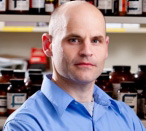DIETZ LAB
 STAFF
STAFFDavid Dietz, Ph.D. This is sample text. This is sample text. This is sample text. This is sample text. This is sample text. This is sample text. This is sample text. This is sample text. This is sample text. This is sample text. This is sample text. This is sample text. This is sample text. This is sample text. This is sample text. Employee Name This is sample text. This is sample text. This is sample text. This is sample text. This is sample text. This is sample text. This is sample text. This is sample text. This is sample text. This is sample text. This is sample text. This is sample text. This is sample text. This is sample text. This is sample text. Employee Name This is sample text. This is sample text. This is sample text. This is sample text. This is sample text. This is sample text. This is sample text. This is sample text. This is sample text. This is sample text. This is sample text. This is sample text. This is sample text. This is sample text. This is sample text. Employee Name This is sample text. This is sample text. This is sample text. This is sample text. This is sample text. This is sample text. This is sample text. This is sample text. This is sample text. This is sample text. This is sample text. This is sample text. This is sample text. This is sample text. This is sample text. Employee Name This is sample text. This is sample text. This is sample text. This is sample text. This is sample text. This is sample text. This is sample text. This is sample text. This is sample text. This is sample text. This is sample text. This is sample text. This is sample text. This is sample text. This is sample text.